## Examples Using Wavelet Packet Tree Objects

You can use command line functions, the Wavelet Analyzer app, or you can mix both of them to work with wavelet packet trees (WPTREE objects). The most useful commands are

We can see some of these features in the following examples.

### plot and wpviewcf

```load noisbump x = noisbump; t = wpdec(x,3,'db2'); fig = plot(t); ```

Click on node 7.Change Node Action from Visualize to Split-Merge and merge the second node.```% From the command line, you can get the new tree. newt = plot(t,'read',fig); % The first argument of the plot function in the last command % is dummy. Then the general syntax is: % newt = plot(DUMMY,'read',fig); % where DUMMY is any object parented by an NTREE object. % DUMMY can be any object constructor name, which returns % an object parented by an NTREE object. For example: % newt = plot(ntree,'read',fig); % newt = plot(dtree,'read',fig); % newt = plot(wptree,'read',fig); % From the command line you can modify the new tree, % then plot it. newt = wpjoin(newt,3); fig2 = plot(newt); % Change Node Label from Depth_position to Index and % click the node (3). You get the following figure. ```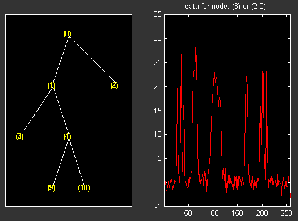```% Using plot(newt,fig), the plot is done in the figure fig, % which already contains a tree object. % You can see the colored wavelet packets coefficients using % from the command line, the wpviewcf function (type help % wpviewcf for more information). wpviewcf(newt,1) % You get the following plot, which contains the terminal nodes % colored coefficients. ```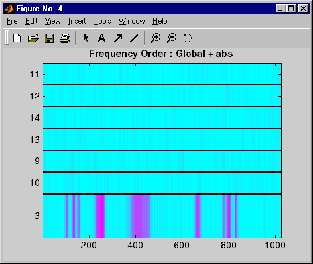```load noisbump x = noisbump; t = wpdec(x,3,'db2'); fig = drawtree(t); % The last command creates a GUI. % The same GUI can be obtained using waveletAnalyzer and: % - clicking the Wavelet Packet 1-D button, % - loading the signal noisbump, % - choosing the level and the wavelet % - clicking the decomposition button. % You get the following figure. ``````% From the app, you can modify the tree. % For example, change Node label from Depth_Position to Index, % change Node Action from Visualize to Split_Merge and % merge the node 2. % You get the following figure. ``````% From the command line, you can get the new tree. newt = readtree(fig); % From the command line you can modify the new tree; % then plot it in the same figure. newt = wpjoin(newt,3); drawtree(newt,fig); ```You can mix previous commands. The GUI associated with the `plot` command is simpler and quicker, but more actions and information are available using portions of the Wavelet Analyzer app related to wavelet packets.

The methods associated with WPTREE objects let you do more complicated actions.

Namely, using `read` and `write` methods, you can change terminal node coefficients.

Let's illustrate this point with the following “funny” example.

### Change Terminal Node Coefficients

```load gatlin2 t = wpdec2(X,1,'haar'); plot(t); % Change Node Label from Depth_position to Index and % click the node (0). You get the following figure. ```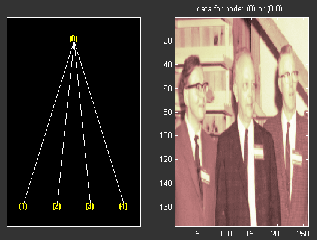```% Now modify the coefficients of the four terminal nodes. newt = t; NBcols = 40; for node = 1:4 cfs = read(t,'data',node); tmp = cfs(1:end,1:NBcols); cfs(1:end,1:NBcols) = cfs(1:end,end-NBcols+1:end); cfs(1:end,end-NBcols+1:end) = tmp; newt = write(newt,'data',node,cfs); end plot(newt) % Change Node Label from Depth_position to Index and % click on the node (0). You get the following figure. ```You can use this method for a more useful purpose. Let's see a denoising example.

### Thresholding Wavelet Packets

```load noisbloc x = noisbloc; t = wpdec(x,3,'sym4'); plot(t); % Change Node Label from Depth_position to Index and % click the node (0). You get the following plot. ```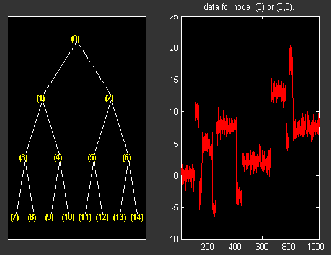```% Global thresholding. t1 = t; sorh = 'h'; thr = wthrmngr('wp1ddenoGBL','penalhi',t); cfs = read(t,'data'); cfs = wthresh(cfs,sorh,thr); t1 = write(t1,'data',cfs); plot(t1) % Change Node Label from Depth_position to Index and % click the node (0). You get the following plot. ``````% Node by node thresholding. t2 = t; sorh = 's'; thr(1) = wthrmngr('wp1ddenoGBL','penalhi',t); thr(2) = wthrmngr('wp1ddenoGBL','sqtwologswn',t); tn = leaves(t); for k=1:length(tn) node = tn(k); cfs = read(t,'data',node); numthr = rem(node,2)+1; cfs = wthresh(cfs,sorh,thr(numthr)); t2 = write(t2,'data',node,cfs); end plot(t2) % Change Node Label from Depth_position to Index and % click the node (0). You get the following plot. ```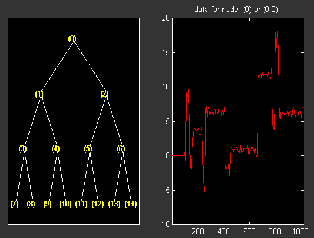##### SupportGet trial now